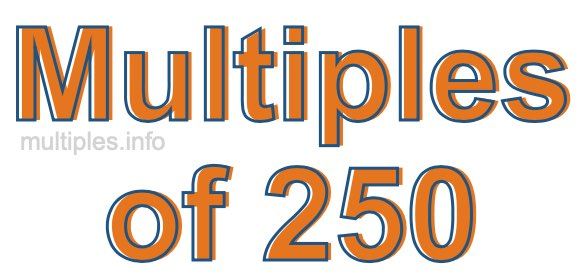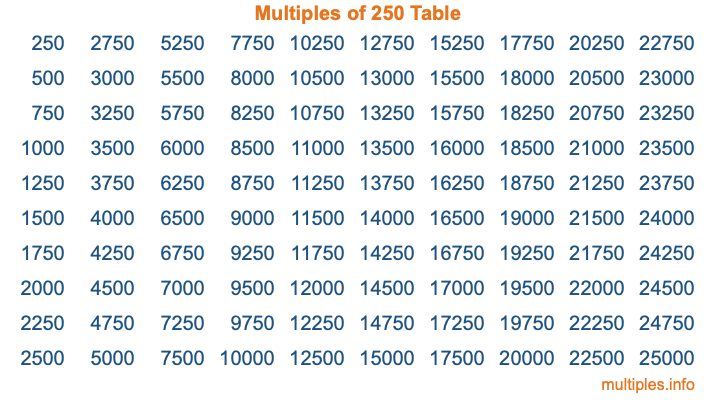Multiples of 250Welcome to the Multiples of 250 page. Here we will first teach you everything you will ever need to know about the multiples of 250, and then give you a study guide summary of everything we taught you to make sure you remember it all. Use this page to look up facts and learn information about the multiples of 250. This page will make you a multiples of two hundred fifty expert!

Definition of Multiples of 250
Multiples of 250 are all the numbers that when divided by 250 equal an integer. Each of the multiples of 250 are called a multiple. A multiple of 250 is created by multiplying 250 by an integer.

Therefore, to create a list of multiples of 250, you start with 1 multiplied by 250, then 2 multiplied by 250, then 3 multiplied by 250, and so on for as long as you want. Thus, the list of the first five multiples of 250 is 250, 500, 750, 1000, and 1250. To see a larger list of multiples of 250, see the printable image of Multiples of 250 further down on this page. We also have a category where you can choose any nth multiple of 250.

Multiples of 250 Checker
The Multiples of 250 Checker below checks to see if any number of your choice is a multiple of 250. In other words, it checks to see if there is any number (integer) that when multiplied by 250 will equal your number. To do that, we divide your number by 250. If the the quotient is an integer, then your number is a multiple of 250.

Is  a multiple of 250?

Least Common Multiple of 250 and ...
A Least Common Multiple (LCM) is the lowest multiple that two or more numbers have in common. This is also called the smallest common multiple or lowest common multiple and is useful to know when you are adding our subtracting fractions. Enter one or more numbers below (250 is already entered) to find the LCM.

Check out our LCM Calculator if you need more details about the Least Common Multiple or if you need the LCM for different numbers for adding and subtraction fractions.

nth Multiple of 250
As we stated above, 250 is the first multiple of 250, 500 is the second multiple of 250, 750 is the third multiple of 250, and so on. Enter a number below to find the nth multiple of 250.

th multiple of 250

Multiples of 250 vs Factors of 250
250 is a multiple of 250 and a factor of 250, but that is where the similarities end. All postive multiples of 250 are 250 or greater than 250. All positive factors of 250 are 250 or less than 250.

Below is the beginning list of multiples of 250 and the factors of 250 so you can compare:

Multiples of 250: 250, 500, 750, 1000, 1250, etc.

Factors of 250: 1, 2, 5, 10, 25, 50, 125, 250

As you can see, the multiples of 250 are all the numbers that you can divide by 250 to get a whole number. The factors of 250, on the other hand, are all the whole numbers that you can multiply by another whole number to get 250.

It's also interesting to note that if a number (x) is a factor of 250, then 250 will also be a multiple of that number (x).

Multiples of 250 vs Divisors of 250
The divisors of 250 are all the integers that 250 can be divided by evenly. Below is a list of the divisors of 250.

Divisors of 250: 1, 2, 5, 10, 25, 50, 125, 250

The interesting thing to note here is that if you take any multiple of 250 and divide it by a divisor of 250, you will see that the quotient is an integer.

Multiples of 250 Table
Below is an image of the first 100 multiples of 250 in a table. The table is in chronological order, column by column. The first column has the first ten multiples of 250, the second column has the next ten multiples of 250, and so on.The Multiples of 250 Table is also referred to as the 250 Times Table or Times Table of 250. You are welcome to print out our table for your studies.

Negative Multiples of 250
Although not often discussed or needed in math, it is worth mentioning that you can make a list of negative multiples of 250 by multiplying 250 by -1, then by -2, then by -3, and so on, to get the following list of negative multiples of 250:

-250, -500, -750, -1000, -1250, etc.

Multiples of 250 Summary
Below is a summary of important Multiples of 250 facts that we have discussed on this page. To retain the knowledge on this page, we recommend that you read through the summary and explain to yourself or a study partner why they hold true.

There are an infinite number of multiples of 250.

A multiple of 250 divided by 250 will equal a whole number.

250 divided by a factor of 250 equals a divisor of 250.

The nth multiple of 250 is n times 250.

The largest factor of 250 is equal to the first positive multiple of 250.

250 is a multiple of every factor of 250.

250 is a multiple of 250.

A multiple of 250 divided by a divisor of 250 equals an integer.

250 divided by a divisor of 250 equals a factor of 250.

Any integer times 250 will equal a multiple of 250.

Multiples of a Number
Here you can get the multiples of another number, all with the same attention to detail as we did for multiples of 250 on this page.

Multiples of
Multiples of 251
Did you find our page about multiples of two hundred fifty educational? Do you want more knowledge? Check out the multiples of the next number on our list!